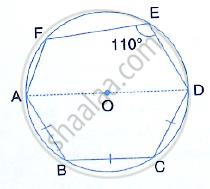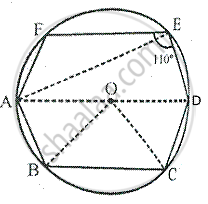Share

# In the Following Figure, Ad is the Diameter of the Circle with Centre O. Chords Ab, Bc and Cd Are Equal. If ∠Def = 110°, Calculate: - Mathematics

Course

#### Question

In the following figure, AD is the diameter of the circle with centre O. chords AB, BC and CD are equal. If ∠DEF = 110°, Calculate:  ∠AEF#### SolutionJoin AE, OB and OC

∵ AOD is the diameter,

∴ ∠AED = 90°                         [Angle in a semi-circle]

But ∠DEF = 110°         [given]

∴ ∠AEF = ∠DEF - ∠AED

= 110° - 90° = 20°

Is there an error in this question or solution?